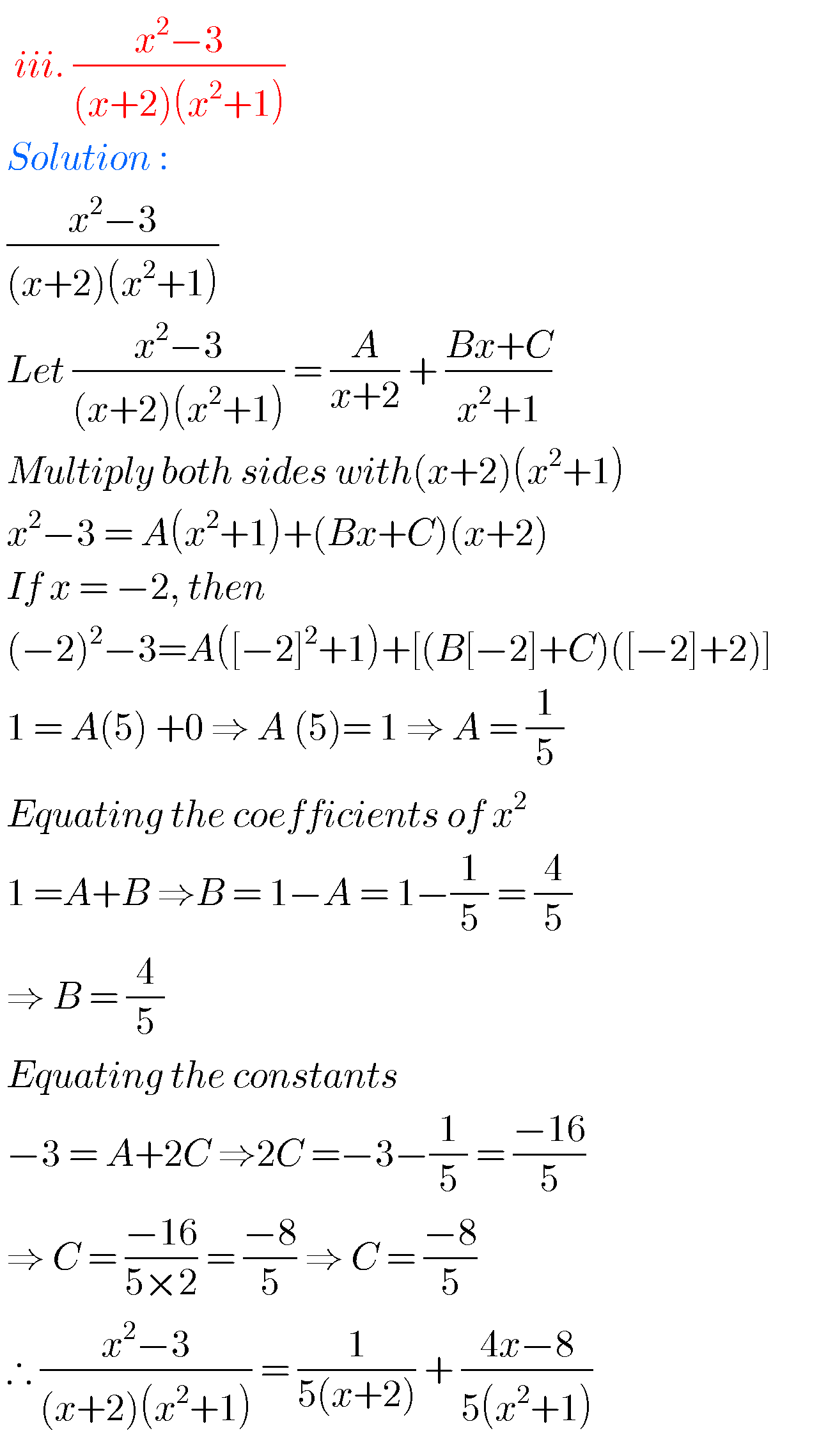## Inter maths solutions for IIA Partial Fractions,exercise 7(a) 7(b) 7(c) 7(d) Intermediate Mathematics 2nd year solutions

Inter maths solutions for IIA Partial Fractions Intermediate second year mathematics text book chapter  exercises 7(a, 7(b), 7(c) and 7(d) solutions are given. These solutions are very easy to understand. Study the text book lesson Partial Fractions very well.  Observe the example problems and solutions given in the textbook. Observe the solutions and try them …